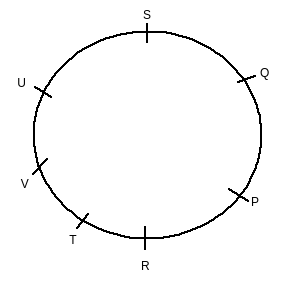# Directions : Study the following information to answer the question: A]         P, Q, R, S, T, U and V are sitting along the circle facing the centre.  B]         T is 2nd to the left of P. C]         R is 3rd to the right of U who is to the immediate left of V. D]         Q is between P and S.   Which of the following is the true statement?     Option 1)   P is between Q and T   Option 2)   R is between P and V         Option 3)   P is 4th to the right of U   Option 4)   U is between V and Q   Option 5)   None of these